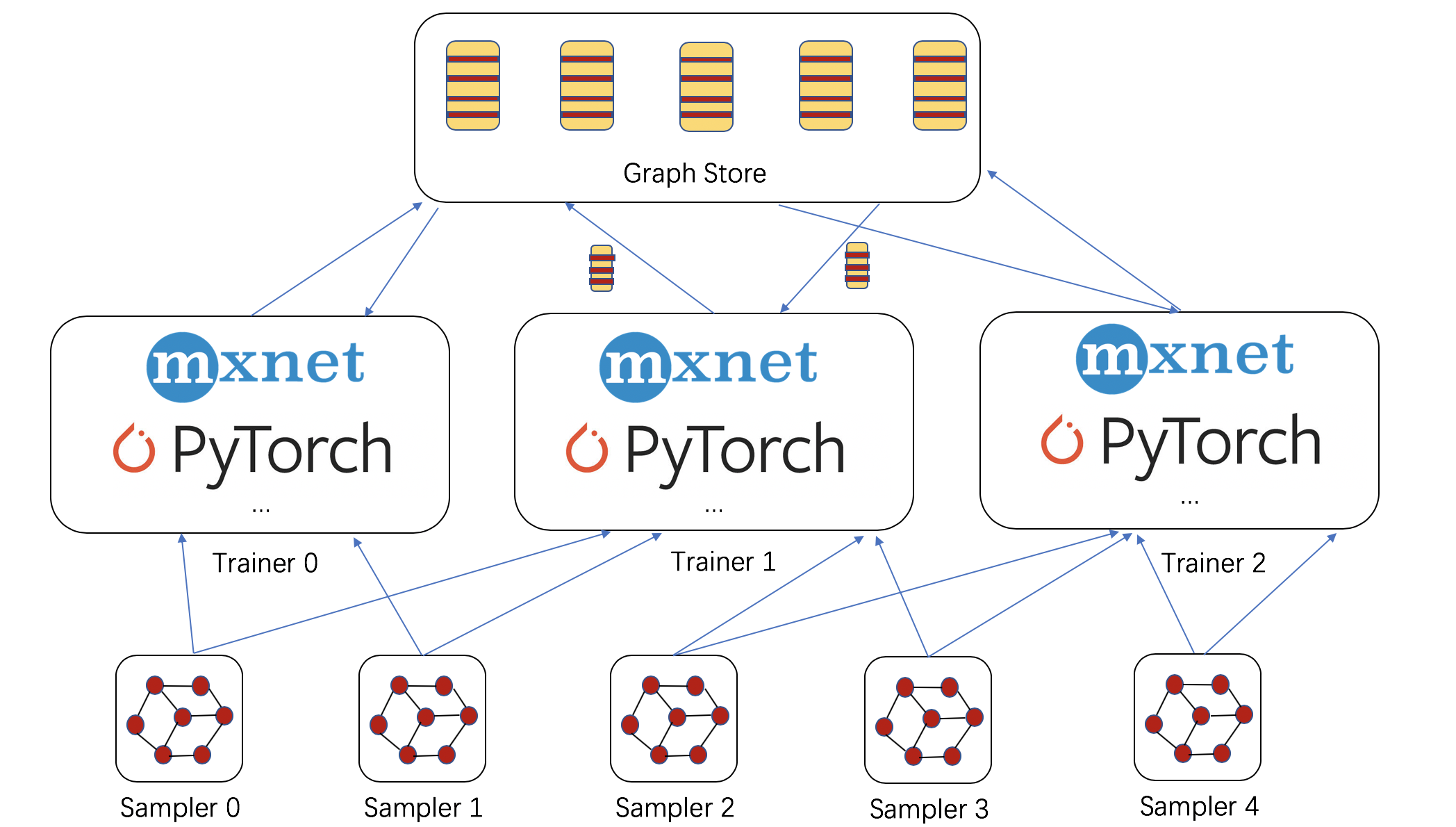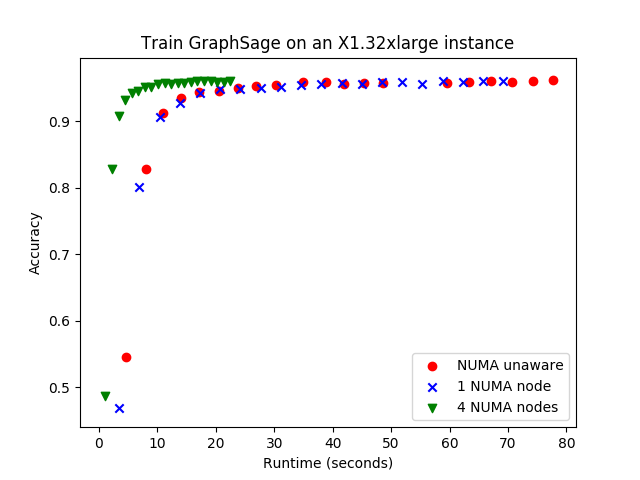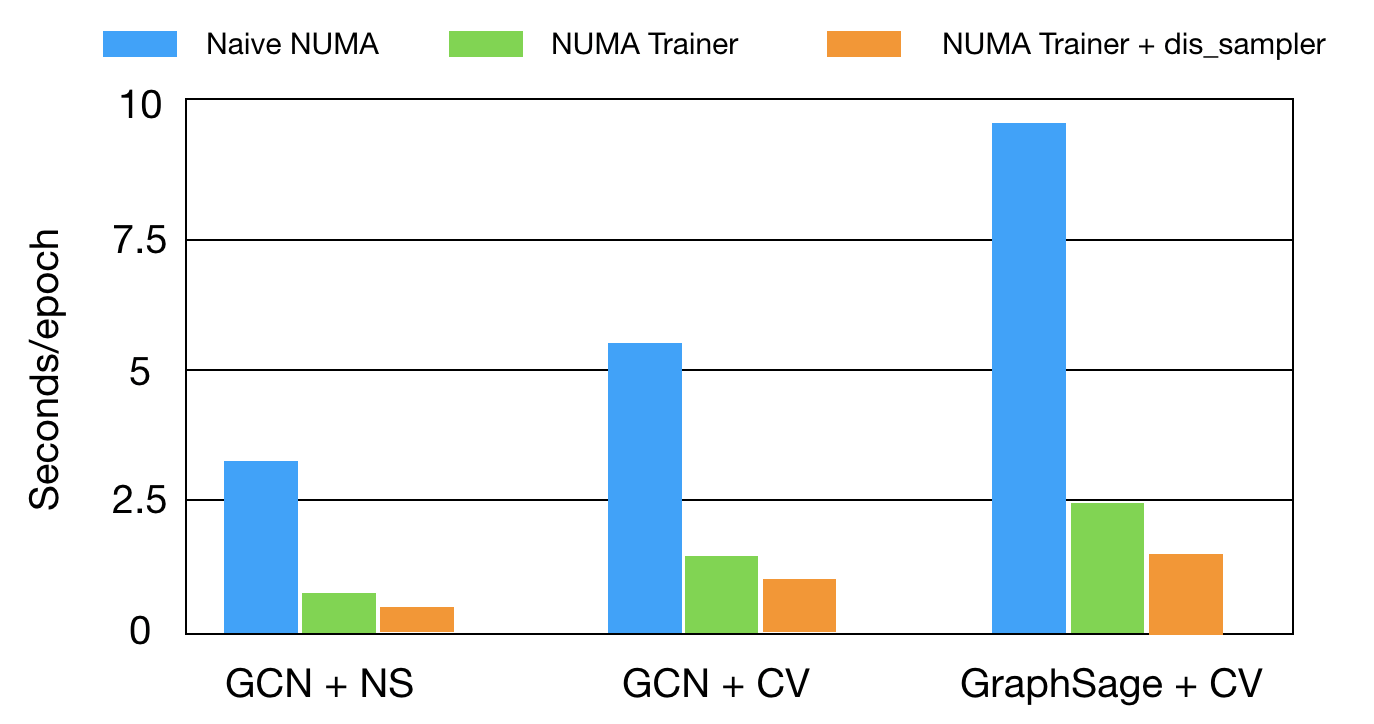# Large-Scale Training of Graph Neural Networks¶

Author: Da Zheng, Chao Ma, Zheng Zhang

In real-world tasks, many graphs are very large. For example, a recent snapshot of the friendship network of Facebook contains 800 million nodes and over 100 billion links. We are facing challenges on large-scale training of graph neural networks.

To accelerate training on a giant graph, DGL provides two additional components: sampler and graph store.

• A sampler constructs small subgraphs (NodeFlow) from a given (giant) graph. The sampler can run on a local machine as well as on remote machines. Also, DGL can launch multiple parallel samplers across a set of machines.

• The graph store contains graph embeddings of a giant graph, as well as the graph structure. So far, we provide a shared-memory graph store to support multi-processing training, which is important for training on multiple GPUs and on non-uniform memory access (NUMA) machines. The shared-memory graph store has a similar interface to DGLGraph for programming. DGL will also support a distributed graph store that can store graph embeddings across machines in the future release.

The figure below shows the interaction of the trainer with the samplers and the graph store. The trainer takes subgraphs (NodeFlow) from the sampler and fetches graph embeddings from the graph store before training. The trainer can push new graph embeddings to the graph store afterward.In this tutorial, we use control-variate sampling to demonstrate how to use these three DGL components, extending the original code of control-variate sampling. Because the graph store has a similar API to DGLGraph, the code is similar. The tutorial will mainly focus on the difference.

## Graph Store¶

The graph store has two parts: the server and the client. We need to run the graph store server as a daemon before training. We provide a script run_store_server.py (link) that runs the graph store server and loads graph data. For example, the following command runs a graph store server that loads the reddit dataset and is configured to run with four trainers.

python3 run_store_server.py --dataset reddit --num-workers 4


The trainer uses the graph store client to access data in the graph store from the trainer process. A user only needs to write code in the trainer. We first create the graph store client that connects with the server. We specify store_type as “shared_memory” to connect with the shared-memory graph store server.

g = dgl.contrib.graph_store.create_graph_from_store("reddit", store_type="shared_mem")


The sampling tutorial shows the detail of sampling methods and how they are used to train graph neural networks such as graph convolution network. As a recap, the graph convolution model performs the following computation in each layer.

$z_v^{(l+1)} = \sum_{u \in \mathcal{N}^{(l)}(v)} \tilde{A}_{uv} h_u^{(l)} \qquad h_v^{(l+1)} = \sigma ( z_v^{(l+1)} W^{(l)} )$

Control variate sampling approximates $$z_v^{(l+1)}$$ as follows:

$\begin{split}\hat{z}_v^{(l+1)} = \frac{\vert \mathcal{N}(v) \vert }{\vert \hat{\mathcal{N}}^{(l)}(v) \vert} \sum_{u \in \hat{\mathcal{N}}^{(l)}(v)} \tilde{A}_{uv} ( \hat{h}_u^{(l)} - \bar{h}_u^{(l)} ) + \sum_{u \in \mathcal{N}(v)} \tilde{A}_{uv} \bar{h}_u^{(l)} \\ \hat{h}_v^{(l+1)} = \sigma ( \hat{z}_v^{(l+1)} W^{(l)} )\end{split}$

In addition to the approximation, Chen et. al. applies a preprocessing trick to reduce the number of hops for sampling neighbors by one. This trick works for models such as Graph Convolution Networks and GraphSage. It preprocesses the input layer. The original GCN takes $$X$$ as input. Instead of taking $$X$$ as the input of the model, the trick computes $$U^{(0)}=\tilde{A}X$$ and uses $$U^{(0)}$$ as the input of the first layer. In this way, the vertices in the first layer does not need to compute aggregation over their neighborhood and, thus, reduce the number of layers to sample by one.

For a giant graph, both $$\tilde{A}$$ and $$X$$ can be very large. We need to perform this operation in a distributed fashion. That is, each trainer takes part of the computation and the computation is distributed among all trainers. We can use update_all in the graph store to perform this computation.

g.update_all(fn.copy_src(src='features', out='m'),
fn.sum(msg='m', out='preprocess'),
lambda node : {'preprocess': node.data['preprocess'] * node.data['norm']})


update_all in the graph store runs in a distributed fashion. That is, all trainers need to invoke this function and take part of the computation. When a trainer completes its portion, it will wait for other trainers to complete before proceeding with its other computation.

The node/edge data now live in the graph store and the access to the node/edge data is now a little different. The graph store no longer supports data access with g.ndata/g.edata, which reads the entire node/edge data tensor. Instead, users have to use g.nodes[node_ids].data[embed_name] to access data on some nodes. (Note: this method is also allowed in DGLGraph and g.ndata is simply a short syntax for g.nodes[:].data). In addition, the graph store supports get_n_repr/set_n_repr for node data and get_e_repr/set_e_repr for edge data.

To initialize the node/edge tensors more efficiently, we provide two new methods in the graph store client to initialize node data and edge data (i.e., init_ndata for node data or init_edata for edge data). What happened under the hood is that these two methods send initialization commands to the server and the graph store server initializes the node/edge tensors on behalf of trainers.

Here we show how we should initialize node data for control-variate sampling. h_i stores the history of nodes in layer i; agg_h_i stores the aggregation of the history of neighbor nodes in layer i.

for i in range(n_layers):
g.init_ndata('h_{}'.format(i), (features.shape, args.n_hidden), 'float32')
g.init_ndata('agg_h_{}'.format(i), (features.shape, args.n_hidden), 'float32')


After we initialize node data, we train GCN with control-variate sampling as below. The training code takes advantage of preprocessed input data in the first layer and works identically to the single-process training procedure.

for nf in NeighborSampler(g, batch_size, num_neighbors,
neighbor_type='in', num_hops=L-1,
seed_nodes=labeled_nodes):
for i in range(nf.num_blocks):
# aggregate history on the original graph
g.pull(nf.layer_parent_nid(i+1),
fn.copy_src(src='h_{}'.format(i), out='m'),
lambda node: {'agg_h_{}'.format(i): node.data['m'].mean(axis=1)})
# We need to copy data in the NodeFlow to the right context.
nf.copy_from_parent(ctx=right_context)
nf.apply_layer(0, lambda node : {'h' : layer(node.data['preprocess'])})
h = nf.layers.data['h']

for i in range(nf.num_blocks):
prev_h = nf.layers[i].data['h_{}'.format(i)]
# compute delta_h, the difference of the current activation and the history
nf.layers[i].data['delta_h'] = h - prev_h
# refresh the old history
nf.layers[i].data['h_{}'.format(i)] = h.detach()
# aggregate the delta_h
nf.block_compute(i,
fn.copy_src(src='delta_h', out='m'),
lambda node: {'delta_h': node.data['m'].mean(axis=1)})
delta_h = nf.layers[i + 1].data['delta_h']
agg_h = nf.layers[i + 1].data['agg_h_{}'.format(i)]
# control variate estimator
nf.layers[i + 1].data['h'] = delta_h + agg_h
nf.apply_layer(i + 1, lambda node : {'h' : layer(node.data['h'])})
h = nf.layers[i + 1].data['h']
# update history
nf.copy_to_parent()


The complete example code can be found here.

After showing how the shared-memory graph store is used with control-variate sampling, let’s see how to use it for multi-GPU training and how to optimize the training on a non-uniform memory access (NUMA) machine. A NUMA machine here means a machine with multiple processors and large memory. It works for all backend frameworks as long as the framework supports multi-processing training. If we use MXNet as the backend, we can use the distributed MXNet kvstore to aggregate gradients among processes and use the MXNet launch tool to launch multiple workers that run the training script. The command below launches our example code for multi-processing GCN training with control variate sampling and it runs 4 trainers.

python3 ../incubator-mxnet/tools/launch.py -n 4 -s 1 --launcher local \
python3 examples/mxnet/sampling/multi_process_train.py \
--graph-name reddit \
--model gcn_cv --num-neighbors 1 \
--batch-size 2500 --test-batch-size 5000 \
--n-hidden 64


It is fairly easy to enable multi-GPU training. All we need to do is to copy data to a right GPU context and invoke NodeFlow computation in that GPU context. As shown above, we specify a context right_context in copy_from_parent.

To optimize the computation on a NUMA machine, we need to configure each process properly. For example, we should use the same number of processes as the number of NUMA nodes (usually equivalent to the number of processors) and bind the processes to NUMA nodes. In addition, we should reduce the number of OpenMP threads to the number of CPU cores in a processor and reduce the number of threads of the MXNet kvstore to a small number such as 4.

import numa
import os
# bind the process to a NUMA node.
# Reduce the number of OpenMP threads to match the number of
# CPU cores of a processor.
else:
# Reduce the number of OpenMP threads in the MXNet KVstore server to 4.


Given the configuration above, NUMA-aware multi-processing training can accelerate training almost by a factor of 4 as shown in the figure below on an X1.32xlarge instance where there are 4 processors, each of which has 16 physical CPU cores. We can see that NUMA-unaware training cannot take advantage of computation power of the machine. It is even slightly slower than just using one of the processors in the machine. NUMA-aware training, on the other hand, takes about only 20 seconds to converge to the accuracy of 96% with 20 iterations.## Distributed Sampler¶

For many tasks, we found that the sampling takes a significant amount of time for the training process on a giant graph. So DGL supports distributed samplers for speeding up the sampling process on giant graphs. DGL allows users to launch multiple samplers on different machines concurrently, and each sampler can send its sampled subgraph (NodeFlow) to trainer machines continuously.

To use the distributed sampler on DGL, users start both trainer and sampler processes on different machines. Users can find the complete demo code and launch scripts in this link and this tutorial will focus on the main difference between single-machine code and distributed code.

For the trainer, developers can easily migrate the existing single-machine sampler code to the distributed setting seamlessly by just changing a few lines of code. First, users need to create a distributed SamplerReceiver object before training:

sampler = dgl.contrib.sampling.SamplerReceiver(graph, ip_addr, num_sampler)


The SamplerReceiver class is used for receiving remote subgraph from other machines. This API has three arguments: parent_graph, ip_address, and number_of_samplers.

After that, developers can change just one line of existing single-machine training code like this:

for nf in sampler:
for i in range(nf.num_blocks):
# aggregate history on the original graph
g.pull(nf.layer_parent_nid(i+1),
fn.copy_src(src='h_{}'.format(i), out='m'),
lambda node: {'agg_h_{}'.format(i): node.data['m'].mean(axis=1)})

...


Here, we use the code for nf in sampler to replace the original single-machine sampling code:

for nf in NeighborSampler(g, batch_size, num_neighbors,
neighbor_type='in', num_hops=L-1,
seed_nodes=labeled_nodes):


All the other parts of the original single-machine code is not changed.

In addition, developers need to write sampling logic on the sampler machine. For neighbor-sampler, developers can just copy their existing single-machine code to sampler machines like this:

sender = dgl.contrib.sampling.SamplerSender(trainer_address)

...

for n in num_epoch:
for nf in dgl.contrib.sampling.NeighborSampler(graph, batch_size, num_neighbors,
neighbor_type='in',
shuffle=shuffle,
num_workers=num_workers,
num_hops=num_hops,
seed_nodes=seed_nodes):
sender.send(nf, trainer_id)
# tell trainer I have finished current epoch
sender.signal(trainer_id)


The figure below shows the overall performance improvement of training GCN and GraphSage on the Reddit dataset after deploying the optimizations in this tutorial. Our NUMA optimization speeds up the training by a factor of 4. The distributed sampling achieves additional 20%-40% speed improvement for different tasks.## Scale to giant graphs¶

Finally, we would like to demonstrate the scalability of DGL with giant synthetic graphs. We create three large power-law graphs with RMAT. Each node is associated with 100 features and we compute node embeddings with 64 dimensions. Below shows the training speed and memory consumption of GCN with neighbor sampling.

#Nodes

#Edges

Time per epoch (s)

Memory (GB)

5M

250M

4.7

8

50M

2.5B

46

75

500M

25B

505

740

We can see that DGL can scale to graphs with up to 500M nodes and 25B edges.

Total running time of the script: ( 0 minutes 0.000 seconds)

Gallery generated by Sphinx-Gallery